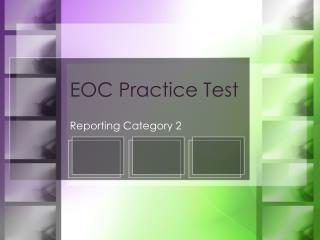DownloadDownload PresentationEOC Practice Test

# EOC Practice Test

Download Presentation## EOC Practice Test

- - - - - - - - - - - - - - - - - - - - - - - - - - - E N D - - - - - - - - - - - - - - - - - - - - - - - - - - -
##### Presentation Transcript

1. EOC Practice Test Reporting Category 2

2. Mathematical Processes Reporting Category 2

3. 1. If x ≠ , which expression is equivalent to ? • 3x – 2 C. –6x2 + x – 6 • B. x + 5 D. –6x2 + 25x – 14

4. Which set of numbers is ordered • from greatest to least? C. D. A. B.

5. A cubic millimeter of human blood contains • about 5 x 106 red blood cells. An adult • human body may contain about 5 x 106 • cubic millimeters of blood. About how many • red blood cells does such a human body • contain? • about 5 x 106 C. about 2.5 x 1012 • about 25 x 106 D. about 2.5 x 1013

6. 4. Which expression is equivalent to ? A. 8x24 B. 8x12 C. 64x12 D. 64x8

7. 5. Which expression is equivalent to ? A. C. B. D. x

8. 6. What is the value of ? A. C. B. D.

9. 7. Which expression is closest to (7.08 x 10–7)(4.033 x 1029)? A. 1.76 x 1022 B. 1.76 x 1023 C. 2.86 x 1022 D. 2.86 x 1023

10. Which statement best describes the values • of the numbers in this set? A. They are between 1 and 1.5. B. They are between 1.5 and 2. C. They are between 2 and 2.5. D. They are between 2.5 and 3.

11. If the value of the variable y is positive, • what is the sum of and ? A. C. B. D.

12. The mass of the Sun is estimated to be • about 1.99 x 1030 kg, and the mass of • Jupiter is estimated to be about • 1.90 x 1027 kg. About how many times • heavier than Jupiter is the Sun? • A. about 2 times • B. about 10 times • C. about 100 times • D. about 1,000 times

13. Which list shows the numbers arranged • from greatest to least? A. B. C. D.

14. 12. Which expression is equivalent to ? A. C. 25x B. 50x D.

15. 13. Which expression is closest to • (5.73 x 10–3)2 ? • A. 0.01146 • B. 3.28 x 10–5 • C. 32,832,900 • D. 3.28 x 10–6

16. 14. Which set of numbers is ordered from least to greatest? A. B. C. D.

17. 15. If the value of the variable x is positive, simplify the expression. A. C. B. D.

19. 1. If x ≠ , which expression is equivalent to ? • 3x – 2 C. –6x2 + x – 6 • B. x + 5 D. –6x2 + 25x – 14

20. Which set of numbers is ordered • from greatest to least? C. D. A. B.

21. A cubic millimeter of human blood contains • about 5 x 106 red blood cells. An adult • human body may contain about 5 x 106 • cubic millimeters of blood. About how many • red blood cells does such a human body • contain? • about 5 x 106 C. about 2.5 x 1012 • about 25 x 106 D. about 2.5 x 1013

22. 4. Which expression is equivalent to ? A. 8x24 B. 8x12 C. 64x12 D. 64x8

23. 5. Which expression is equivalent to ? A. C. B. D. x

24. 6. What is the value of ? A. C. B. D.

25. 7. Which expression is closest to (7.08 x 10–7)(4.033 x 1029)? A. 1.76 x 1022 B. 1.76 x 1023 C. 2.86 x 1022 D. 2.86 x 1023

26. Which statement best describes the values • of the numbers in this set? A. They are between 1 and 1.5. B. They are between 1.5 and 2. C. They are between 2 and 2.5. D. They are between 2.5 and 3.

27. If the value of the variable y is positive, • what is the sum of and ? A. C. B. D.

28. The mass of the Sun is estimated to be • about 1.99 x 1030 kg, and the mass of • Jupiter is estimated to be about • 1.90 x 1027 kg. About how many times • heavier than Jupiter is the Sun? • A. about 2 times • B. about 10 times • C. about 100 times • D. about 1,000 times

29. Which list shows the numbers arranged • from least to greatest? A. B. C. D.

30. 12. Which expression is equivalent to ? A. C. 25x B. 50x D.

31. 13. Which expression is closest to • (5.73 x 10–3)2 ? • A. 0.01146 • B. 3.28 x 10–5 • C. 32,832,900 • D. 3.28 x 10–6

32. 14. Which set of numbers is ordered from least to greatest? A. B. C. D.

33. 15. If the value of the variable x is positive, simplify the expression. A. C. B. D.